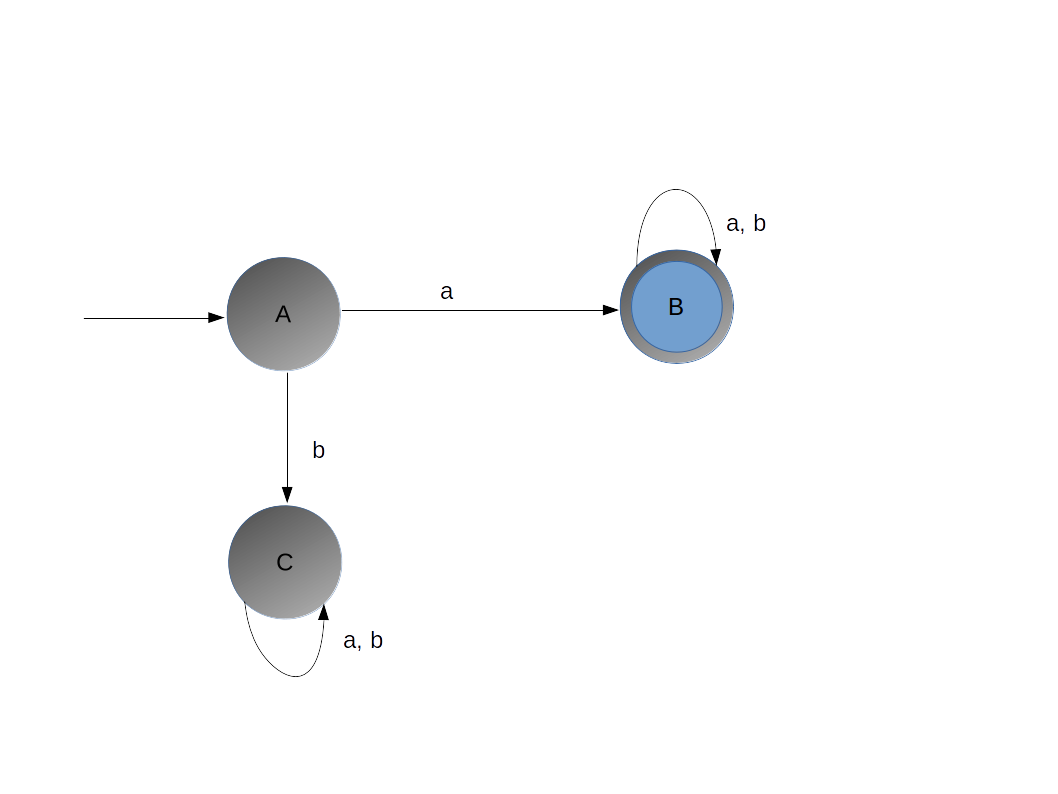# TOC Introduction

Theory of computataion or automata is very important subject in computer science. Any algorithm first goes through this before implementation

### Terminology

We will explain various terms before stating the turorial:
Symbol
: This are english alphabets eg. {a, b, c, d}
String
: Combinatin of alphabets any number of times is called a string. eg. {aa, ab, aaaa, ....}

#### Number of Strings:

If there are m number of alphabets then number of string possible are m^n.

#### Language:

A language is a combination of strings given certain condition:
Example 1 : a language L1 = Set of all strings of length 2 = {aa,ab, bb, ba}
And also we can say that language is finite.

Example 2 : a language L2 = Set of all strings of length 3 = {aaa, bbb, aab, bba, baa, ....}
So total number of strings are 2^3 = 8.
Example 3 : a language L3 = Set of all strings staring with a = {a, aa, aab, aaaaab, ....}
Here number of strings in the language will be infinite.

### Powers of Σ

Let sigma = {a, b}
Σ^1 = Set of all strings of length 1.
Σ^2 = Set of all strings of length 2.
Σ^3 = Set of all strings of length 3.
Σ^n = Set of all strings of length n.

Σ^0 = {∈} // here ∈ shows an empty symbol.

So sigma^* = Σ^0 ∪ Σ^ 1 ∪ Σ^2 ∪ Σ^3...... ∪ Σ^n
So this will be infinite.

L1, L2, L3 ⊆ Σ*
Any Subset of Σ is a language, so how many languages we will get = ∞

### Need of Finite Representaion of Language

We cannot match any strings in language if the language is ˞, So we have curated one finite represenation of the language, called Finite Automata.
And it will tell the membership of the string in the language.
L1 = Set of all strings starting with a over Σ = {a,b}So above, there are three states and the one with double circle is Final State.
And state A is start state.
And state C is dead state (means you cannot reach final state once gotten in dead state).

You can try out any string and check in the automata whether you get to final state or not.
For take any string and scan from left to right for example abba
1. first a came on State A, so you see the diagram and you went to state B.
2. second symbol from string is b, and form diagram we can see that state B goes to B itself on symbol b
3. third symbol from string is b, and form diagram we can see that state B goes to B itself on symbol b
4. fourth symbol from string is a, and form diagram we can see that state B goes to B itself on symbol a
5. State B is final state and the string is also finished. So we will say that string is accepted.

Let's see one string which does not belong to the language
String = baaab
1. first b came on State A, so you see the diagram and you went to state C.
2. second symbol from string is a, and form diagram we can see that state C goes to C itself on symbol b
3. third symbol from string is a, and form diagram we can see that state C goes to C itself on symbol b
4. fourth symbol from string is a, and form diagram we can see that state C goes to C itself on symbol b
5. fifth symbol from string is b, and form diagram we can see that state C goes to C itself on symbol b
6. String is finished and the state is non-final state, so we declare that string does not belong to the language.